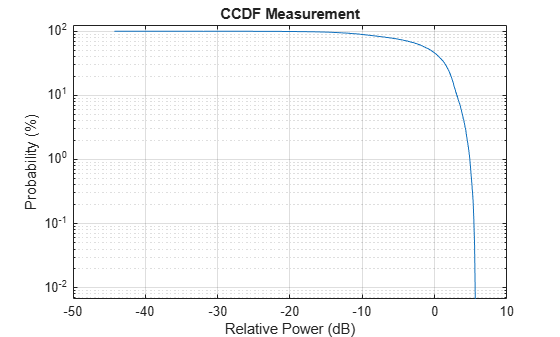# ccdf

Get coordinates of CCDF curves

Since R2022a

## Syntax

``[relpower,prob] = ccdf(meter)``

## Description

example

````[relpower,prob] = ccdf(meter)` returns the coordinates of the CCDF curves.```

## Examples

collapse all

Compute the average power measurement of the generated voltage signal.

```x = complex(rand(15000,1)-0.2,rand(15000,1)-0.2); pm = powermeter(ComputeCCDF=true); averagePower = pm(x); % power in dB```

Plot the computed coordinates of the CCDF curve.

```[relpower,prob] = ccdf(pm); semilogy(relpower,prob) xlabel('Relative Power (dB)'); ylabel('Probability (%)'); ylim([0 125]); grid on; title('CCDF Measurement');```## Input Arguments

collapse all

Power meter, specified as a `powermeter` System object™.

## Output Arguments

collapse all

Relative power in dB, returned as an N-by-M matrix. M equals the number of columns in the signals provided and N equals ceil(`PowerRange`/`PowerResolution`) + 1.

Data Types: `double`

Probability in percentage, returned as a matrix. The probability that the instantaneous signal power of the jth channel is above its average signal power by `relpower`(i,j), in dB, is `prob`(i)/100.

Data Types: `double`

## Version History

Introduced in R2022a